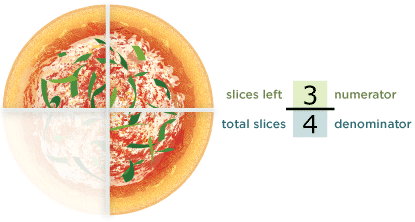# What's a Fraction?: Activity 1 of 3

## Directions

A fraction describes part of a whole. Let’s look at a pie cut into 4 equal slices. The pie is the whole and we’ve divided the whole into 4 equal parts.

If 1 slice is eaten and 3 are left, what fraction of the pie is left?

## Math11_A1_Q1-10.pngThe bottom number, known as the denominator, shows the total number of slices. The top number, or numerator, shows the number of parts we’re talking about.

Question 1 of 10